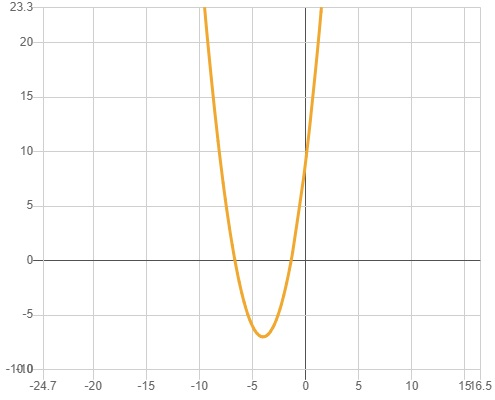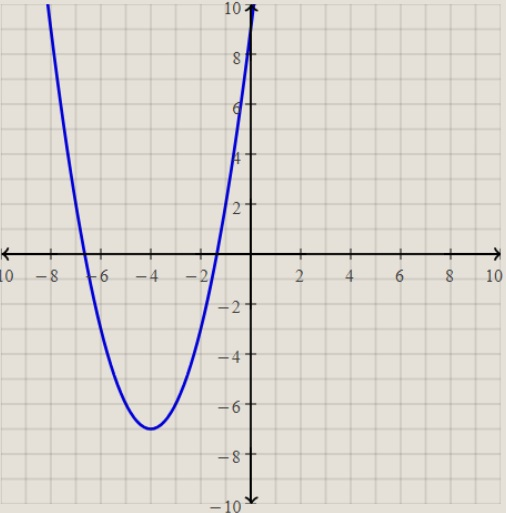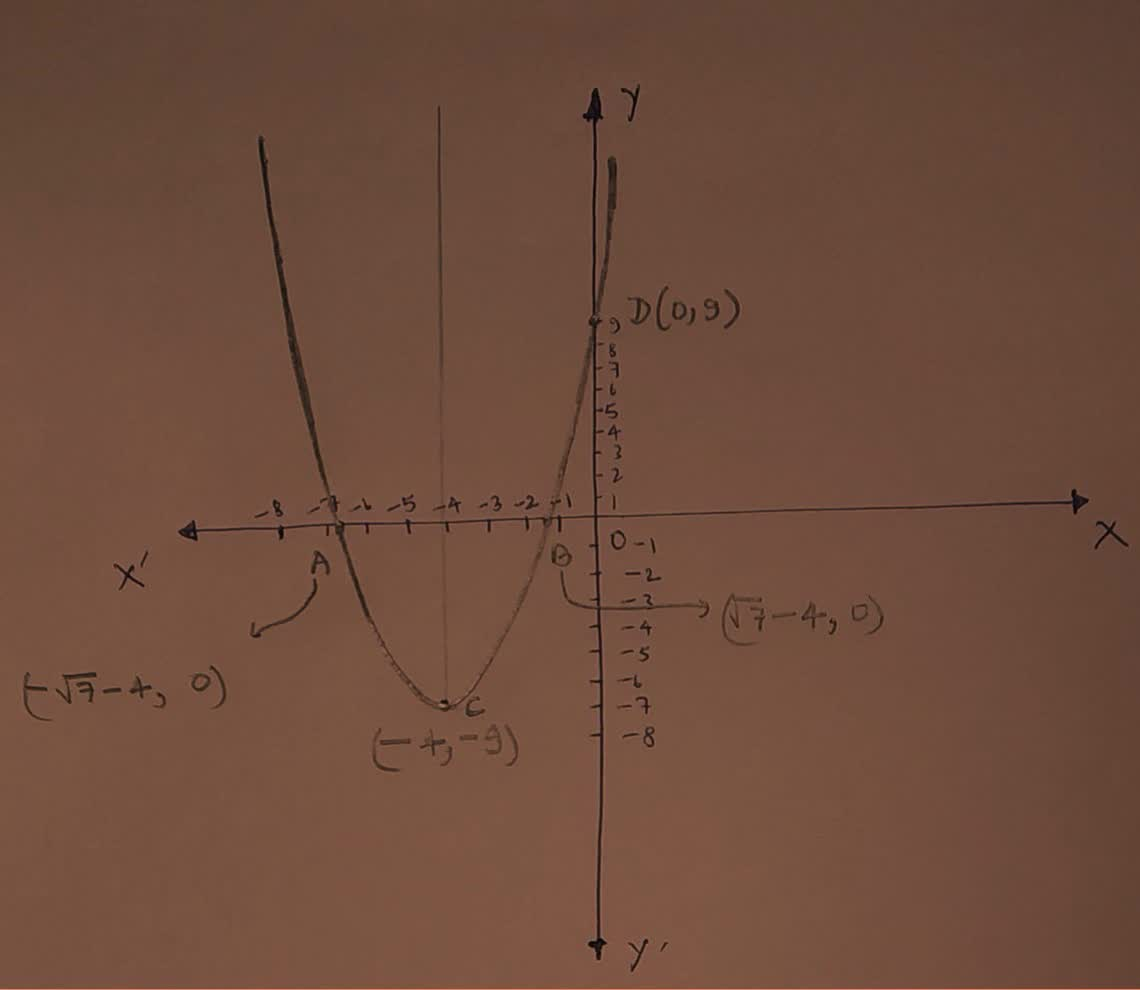Sapewa

2021-12-27

$y={\left(x+4\right)}^{2}-7$
a) Determine the x-intercepts of the quadratic equation in both approximate and exact form
b) Determine the vertex point.
c) Determine the y-intercept.
d) Graph the parabola.Fasaniu

Expert

Step 1
Solution: $y={\left(x+4\right)}^{2}-7$
${\left(x+4\right)}^{2}=y+7$
1) By substituting $y=0$, the x intercept can be achieved.
${\left(x+4\right)}^{2}=0+7$
${\left(x+4\right)}^{2}=7$
$x+4=±\sqrt{7}$
$x=±\sqrt{7}-4$
Because of this, x intercepts are  $\sqrt{7}-4$ and $-\sqrt{7}-4$
2) contrasting it with the fundamental parabola equation
${\left(x-h\right)}^{2}=4a\left(y-k\right)$
${\left(x+4\right)}^{2}=\left(y+7\right)$

Therefore vertex is
Step 2
3) You can get the y intercept by changing $x=0$
$y={\left(x+4\right)}^{2}-7$
$y={\left(0+4\right)}^{2}-7$
$y=16-7=9$
4) ${\left(x-h\right)}^{2}=4a\left(y-k\right)$
${\left(x+4\right)}^{2}=\left(y+7\right)$
$\therefore 4a=1$
$a=\frac{1}{4}$
Therefore four of the parabola is of
Also $y={\left(x+4\right)}^{2}-7$
$y={x}^{2}+16+8x-7$
$y={x}^{2}+8x+9$
Coefficient of ${x}^{2}=1⇒\text{Parabola}$ opens up wards.temzej9

Expert

Step 1
Use binomial theorem ${\left(a+b\right)}^{2}={a}^{2}+2ab+{b}^{2}$ to expand ${\left(x+4\right)}^{2}$
$y={x}^{2}+8x+16-7$
Subtract 7 from 16 to get 9.
$y={x}^{2}+8x+9$
Swap sides so that all variable terms are on the left hand side.
${x}^{2}+8x+9=y$
Subtract y from both sides.
${x}^{2}+8x+9-y=0$
This equation is in standard form: $a{x}^{2}+bx+c=0$
Substitute 1 for a, 8 for b, and $9-y$ for c in the quadratic formula, $\frac{-b±\sqrt{{b}^{2}-4ac}}{2a}$
$x=\frac{-8±\sqrt{{8}^{2}-4\left(9-y\right)}}{2}$
Square 8.
$x=\frac{-8±\sqrt{64-4\left(9-y\right)}}{2}$
Multiply -4 times $9-y$.
$x=\frac{-8±\sqrt{64+4y-36}}{2}$
Add 64 to $-36+4y$.
$x=\frac{-8±\sqrt{4y+28}}{2}$
Take the square root of $28+4y$.
1) $x=\frac{-8±2\sqrt{y+7}}{2}$
Now solve the equation (1) when $±$ is plus. Add -8 to $2\sqrt{7+y}$
$x=\frac{2\sqrt{y+7}-8}{2}$
Divide $-8+2\sqrt{7+y}$ by 2
$x=\sqrt{y+7}-4$
When $±$ is minus. Subtract $2\sqrt{7+y}$ by
$x=-\sqrt{y+7}-4$
The equation is now solved
$x=\sqrt{y+7}-4$
$x=-\sqrt{y+7}-4$karton

Expert

Step 1
a) Write the equation in standard form
$-{x}^{2}-8x+y-9=0$
Step 2
Substitute the values of a, b, and c into the quadratic formula
$x=\frac{-\left(-8\right)-\sqrt{\left(-8{\right)}^{2}-4\left(-1\right)\left(y-9\right)}}{2\left(-1\right)}$ or $x=\frac{-\left(-8\right)+\sqrt{\left(-8{\right)}^{2}-4\left(-1\right)\left(y-9\right)}}{2\left(-1\right)}$
Step 3
Simplify the discriminant

Step 4
Simplify

Step 5
b) Let's focus on:
$\left(x+4{\right)}^{2}-7$
Expand the expression
${x}^{2}+8x+16-7$
Step 6
Group like terms together
${x}^{2}+8x+\left(16-7\right)$
Step 7
${x}^{2}+8x+9$
c) Parabola equation in polynomial form
The vertex ofan up-down facing parabola of the form $y=a{x}^{2}+bx+c$ is ${x}_{v}=-\frac{b}{2a}$
Rewrite $y=\left(x+4{\right)}^{2}-7$ in the form $y=a{x}^{2}+bx+c$
$y={x}^{2}+8x+9$
The parabola params are:
$a=1,\phantom{\rule{1em}{0ex}}b=8,\phantom{\rule{1em}{0ex}}x=9$
${x}_{v}=-\frac{b}{2a}$
${x}_{v}=-\frac{8}{2×1}$
Simplify
${x}_{v}=-4$
Plug in ${x}_{v}=-4$ to find the ${y}_{v}$ value
${y}_{v}=-7$
Therefore the parabola vertex is
(-4, -7)
If a<0, then the vertex is a maximum value
If Pa>0, then the vertex is a minimum value
Minimum (-4, -7)
Step 8Do you have a similar question?